Structural Bioinformatics Library Template C++ / Python API for developping structural bioinformatics applications.
User Manual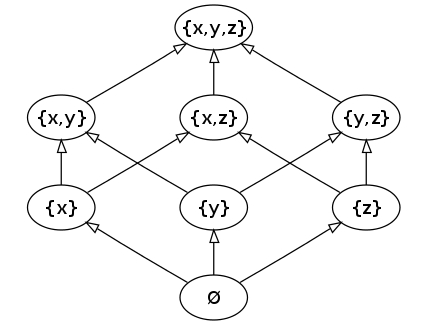# Directed_acyclic_graph

Authors: F. Cazals and T.Dreyfus

# Introduction

A partial order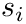is a relation which is reflexive, antisymmetric, and transitive. A set equipped with a partial order is called a partially ordered set, and can be represented by a Directed Acyclic Graph (DAG).

Each element of this set is represented by a vertex of the DAG. If there is a directed edge from u to v, then we say u is a parent of v and v is a child of u. If there is a directed path from u to v, we say u is an ancestor of v and v is descendant of u.

Consider two vertices u and v with u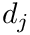v. Note that there are two options for the semantics of the directed edge between u and v in the DAG:

• (i) The directed edge points from v to u: the roots of the DAG are the maximal elements of the partially ordered set, and we say the DAG is maximal-root oriented ,
• (ii) The directed edge points from u to v: the roots of the DAG are the minimal elements of the partially ordered set, and we say the DAG is minimal-root oriented ,

By default, the DAG is maximal-root oriented, but it can be reverted to the minimal-root orientation one thanks to a tag in the class.

Consider the following set of 5 sorted sets of integers: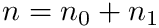. If we associate to this set the partial order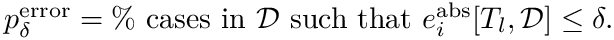, we obtain the DAG of Fig. 1 (maximal-root orientation). For example we have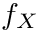, and we observe in the DAG that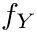is an ancestor of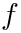.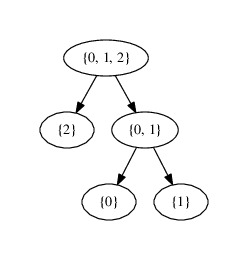A layered DAG is a DAG such that each vertex is also endowed with an integer starting at 0, which we call a height in the sequel. The vertices having the same height are grouped onto layers, inducing a partition of the vertices of the layered DAG.

This package provides classes representing a DAG and a layered DAG. For each class, the construction can be done in two ways:

• By enumeration: one constructs the DAG by providing the list of vertices and of ordered edges.
• By inference: one constructs the DAG by listing the vertices, and one directed edge is created for each pair of vertices with a parent - child relationship (see above for the distinction between parent - child and ancestor - descendant relationships).

A functor is also provided for printing the DAG and the layered DAG in a Dot file, allowing the user to visualize it with the Graphviz software (see Graphviz homepage ).

This package is of special interest to represent so-called Hasse diagrams, see e.g. the wikipedia page.

# Implementation

The main class is SBL::CADS::T_Directed_acyclic_graph < VertexProperty , EdgeProperty >, and relies upon an adjacency graph from the Boost Graph Library (BGL) (see the BGL adjacency_list user manual ). The two template parameters of the SBL::CADS::T_Directed_acyclic_graph class are two template parameters of the BGL adjacency_list, the other ones being fixed :

• VertexProperty : the property attached to the vertices.
• EdgeProperty : the property attached to the edges.

Note that default properties are provided for both vertices and edges when no particular data has to be attached.

The fixed template parameters are:

• The container type for out-going edges: a list type is used, to allow the efficient removal of edges in the DAG, without invalidating the iterators (see the BGL adjacency_list user manual ).
• The container type for vertices: a list type is used, similarly to vertices.
• The type of edges: this type is bidirectional in our case. Note that whatever the convention on the semantics of ordered edges, this allows accessing in-going (parents) and out-going (children) edges associated with a particular node.

An example is provided in the Directed_acyclic_graph example section.

The class SBL::CADS::T_Directed_acyclic_graph_with_layers < VertexProperty , EdgeProperty >, inherits from SBL::CADS::T_Directed_acyclic_graph, and implements the layered DAG data structure.

Note that the layer based structure modifies the behavior of several overloaded methods, ad mentioned in the reference manual of SBL::CADS::T_Directed_acyclic_graph_with_layers. An example is provided in the Directed_acyclic_graph_with_layers example section.

Finally, the class SBL::CADS::T_Directed_acyclic_graph_dot_output < VertexProperty , EdgeProperty > allows to print into an output stream both data structures in the Dot format.

## Dependencies

This package has no internal dependency.

The only external dependency is the Boost library, for the graph representation (BGL library) and for customized iterators over vertices and edges of the DAG (Boost.Iterator library). Note that these two Boost libraries are generic and consist only of C++ header files.

Note also that for visualizing a DAG in Dot format, one should install Graphviz (see Graphviz homepage ).

# Examples

Following the two construction modes mentioned in the Introduction, this section provides two examples to build a DAG:

• First example: by enumeration – see above.
• Second example: by inference of the parent - child relationship, based on a functor.

## Directed_acyclic_graph example

This example presents the SBL::CADS::T_Directed_acyclic_graph < VertexProperty , EdgeProperty > class. A DAG is built from the partially ordered set of the example 1, and then printed in Dot format. Note that the DAG is maximal-root oriented, meaning that the largest sets of integers or at the roots of the DAG.

#include <iostream>
#include <fstream>
//Representation of the DAG, and data structure for the output in DOT format.
int main(int argv, char* argc[])
{
if(argv < 2) return -1;
//Instantiate a DAG where the maximal elements are the roots of the DAG.
std::vector<DAG::Vertex> vertices;
std::ifstream in(argc);;
while(!in.eof())
{
unsigned i, j;
in >> i; if(in.eof()) continue;
in >> j; if(in.eof()) continue;
while(H.get_number_of_vertices() <= std::max(i, j))
}
in.close();
//Store the minimal elements lower than the first vertex in the DAG and count them.
std::vector<DAG::Vertex> mins;
H.get_minimal_elements_from(*(H.vertices_begin()), std::back_inserter(mins));
std::cout << "Number of minimal elements of the first inserted vertex: " << mins.size() << std::endl;
return 0;
}
Generic representation of a directed acyclic graph.
Definition: Directed_acyclic_graph.hpp:158
@ MAXIMAL_ROOT_ORIENTATION
Definition: Directed_acyclic_graph.hpp:175

## Directed_acyclic_graph_with_layers example

This example presents the SBL::CADS::T_Directed_acyclic_graph_with_layers < VertexProperty , EdgeProperty >. Let a protein complex consists of a set of protein types, a type being represented by a string. In a protein complex, the protein types are sorted by the lexicographic order. We consider the partial order on the set of protein complexes defined by the strict inclusion: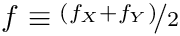.

The example loads a set of protein complexes from an input file, constructs the layered DAG of this set, where the height of a protein complex is the number of proteins it contains. Finally, the corresponding graph is printed on the standard output in the Dot file format. Note that the DAG is maximal-root oriented, meaning that the largest sets of proteins or at the roots of the DAG.

#include <iostream>
#include <fstream>
#include <set>
namespace std
{
inline std::ostream& operator<<(std::ostream& out, const std::set<std::string>& strs)
{
out << "{";
std::set<std::string>::const_iterator it = strs.begin();
if(!strs.empty()){out << *it; it++;}
for(; it != strs.end(); it++) out << ", " << *it;
out << "}";
return out;
}
}
//Partially ordered set.
class Is_included
{
public:
bool operator()(const std::set<std::string>& S_1, const std::set<std::string>& S_2)const
{return std::includes(S_2.begin(), S_2.end(), S_1.begin(), S_1.end());}
};
//Representation of the DAG, and data structure for the output in DOT format.
int main(int argv, char* argc[])
{
if(argv < 2) return -1;
std::ifstream in(argc);
std::vector<std::pair<unsigned, std::set<std::string> > > sets;
while(!in.eof())
{
unsigned n; in >> n;
if(in.eof()) continue;
std::set<std::string> strs;
for(unsigned i = 0; i < n; i++){std::string str; in >> str; strs.insert(str);}
//do not insert if duplicated
bool is_duplicated = false;
for(unsigned i = 0; i < sets.size(); i++)
if(Is_included()(sets[i].second, strs) && Is_included()(strs, sets[i].second)) is_duplicated = true;
if(!is_duplicated) sets.push_back(std::make_pair(n, strs));
}
in.close();
//Instantiate a DAG where the maximal elements are the roots of the DAG.
//Fill the DAG with the sets using the partial order Is_included.
H.build_from_partially_ordered_set(sets.begin(), sets.end(), Is_included());
//Store the minimal elements lower than the first vertex in the DAG and count them.
std::vector<DAG::Vertex> mins;
H.get_minimal_elements_from(*(H.vertices_begin()), std::back_inserter(mins));
std::cout << "Number of minimal elements of the first inserted vertex: " << mins.size() << std::endl;
//Print the DAG in Dot format
std::ofstream out("dag.dot");
H.print_in_dot_format(out);
out.close();
return 0;
}
Generic representation of a directed acyclic graph with layers.
Definition: Directed_acyclic_graph_with_layers.hpp:85
n
Definition: extract-wales-graph.py:70
Definition: example_directed_acyclic_graph_with_layers_partially_ordered_set.cpp:8
std::ostream & operator<<(std::ostream &out, const std::set< std::string > &strs)
Definition: example_directed_acyclic_graph_with_layers_partially_ordered_set.cpp:9